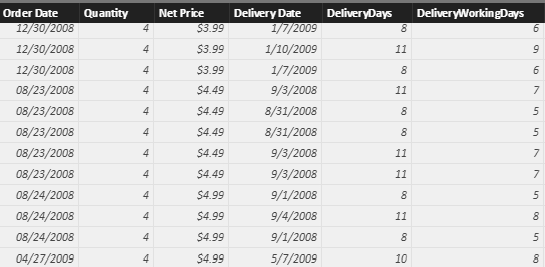The example includes a Sales table containing order and delivery dates. DAX can compute the difference between two dates by subtracting one from the other. This produces the number of days between the two dates – a task that can be accomplished through a calculated column.How is it possible to compute the difference between the two dates, only computing working days and skipping weekends and holidays? Simple math is no longer useful here, and DAX does not offer a predefined function.

A solution to this scenario requires a date table – more details here – with a specific column, IsWorkingDay, which indicates whether that particular day is a working day or not. The following figure shows an example:The IsWorkingDay column should be added to the existing Date table, otherwise it is necessary to create an ad-hoc date table for this purpose. In the demo file of this article, IsWorkingDay is computed by simply checking if the date is a Saturday or Sunday.

```'Date'[IsWorkingDay] = NOT WEEKDAY( 'Date'[Date] ) IN { 1,7 }
```

Using the IsWorkingDay column, the Sales table can now include a new calculated column which writes as follows:

```Sales[DeliveryWorkingDays] =
CALCULATE(
COUNTROWS ( 'Date'),
DATESBETWEEN ( 'Date'[Date],  Sales[Order Date], Sales[Delivery Date] – 1 ),
'Date'[IsWorkingDay] = TRUE,
ALL ( Sales )
)
```

The function uses the DATESBETWEEN function, which returns a table with all the dates between the boundaries – Order Date and Delivery Date in the example. The result of DATESBETWEEN is further restricted by CALCULATE, which applies the second filter to only consider working days.

Once the two filters are applied by CALCULATE, the Date table specifically filters the working days between order and delivery. Then, the COUNTROWS function returns the number of working days in the DeliveryWorkingDay column, as shown in this final figure:DATESBETWEENReturns the dates between two given dates.

`DATESBETWEEN ( <Dates>, <StartDate>, <EndDate> )`

CALCULATEContext transition

Evaluates an expression in a context modified by filters.

`CALCULATE ( <Expression> [, <Filter> [, <Filter> [, … ] ] ] )`

COUNTROWSCounts the number of rows in a table.

`COUNTROWS ( [<Table>] )`